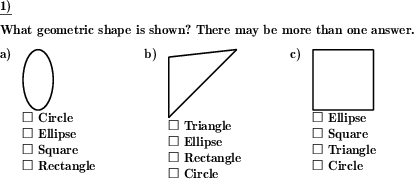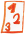Math worksheetsCustom math worksheets at your fingertips

# Details for problem "Name the 2d geometric shape"

Quickname: 3344

## Summary

Name the two dimensional flat geometric shape shown

## Example## Description

A geometric shape is shown. The name has the be determined. Actually, more than one answer may be correct.

For each problem, a single shape is shown. Possible shapes are:

- rectangle
- square
- parallelogram
- rhombus
- trapezoid

Other shapes:

- circle
- ellipse
- triangle

For every shape a selection can be made whether it may occur.

The answer can be requested or presented in the following ways:

- no possible answer is given, the problem is presented as an open question

- multiple possible answers are presented in a multiple choice style. Only one answer is correct.

- multiple possible answers are presented in a multiple choice style. More than one answer may be correct. There is a hint in the problem statement that this may be the case.

- multiple possible answers are presented in a multiple choice style. More than one answer may be correct. There is no hint in the problem statement that this may be the case.

The number of problems is selectable.

Download free worksheets for this math problem here. There is a second sheet with the solutions. Just click on the respective link.Worksheet 1Solution sheetWorksheet 2Solution sheetWorksheet 3Solution sheet

If you can not see the solution sheets for download, they may be filtered out by an ad blocker that you may have installed. If this is the case, please allow ads for this page and reload the page. The solution sheets will then reappear.

• Do the sample worksheets do not really fit?
• Do you need more math worksheets, with a different level of difficulty?
• Would you like to combine different problems on a worksheet and adjust them to your needs?
• As a teacher, you can put together your own worksheets using the automatically generated math problems provided.
With a free initial credit, you can start creating your own math worksheets in a few minutes.

It does not cost anything to try! Register here, to create custom worksheets now!

## Customization options for this problem

ParameterPossible values
Number of problems1, 2, 3, 4, 5, 6
Rectanglesno, rectangle, square, square,rectangle
TriangleYes, No
Ellipsesno, ellipse, circle, circle,ellipse
Rhombusesno, parallelogram, rhombus, parallelogram,rhombus
TrapezoidYes, No
Form of question/answeropen question, multiple choice, mult.choice, mult.correct, mc, no hint on # answers

Deutsche Version dieser Aufgabe
These informational pages with samples describe math problems that can be combined on custom math worksheets with solutions for home and school use.